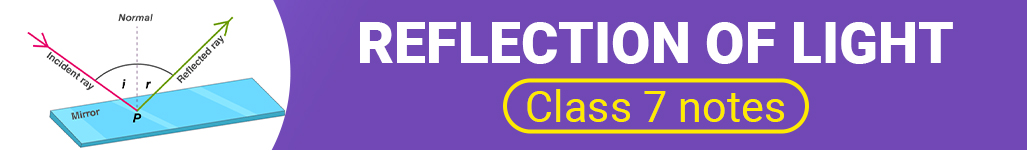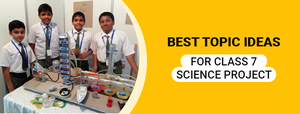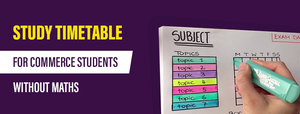Reflection of Light- Class 7 Notes - Study24x7New to Study24X7 ?

# Reflection of Light- Class 7 Notes

Updated on 28 January 2021CBSE CLASS 7
Updated on 28 January 2021In Physics, Reflection of Light is an important chapter where students learn how the light waves travel from one medium to another. Reflection is basically the change in the direction of light waves. The law of reflection includes specular reflection. One example of specular reflection is the mirror.

Sometimes, reflection also causes echoes. Reflection can also be seen on the surface of rivers, lakes and other water bodies. Well, talking in terms of experiments and projects, the reflection of light can be experimented by various objects. Now, let us have a look at reflection and diffusion of light which is included in the light lesson plan for class 7th

Reflection of Light can be defined as the process where the parallel light wave is reflected as the parallel beam in one direction. The examples include plane mirrors or polished metals. A plane mirror produces a reflection of light in the sense that the angle of incidence and the angle of reflection are the same.In diffuse reflection of light, the light waves are reflected in different directions. The incident rays do not remain parallel, rather they are scattered in various other directions. The diffuse reflection of light takes place through rough surfaces like paper, chalk, cardboard, chair and unpolished metals. In this case, the angle of incidence and the angle of reflection are different.In Figure 1, the incident rays and reflected rays are the same leading to the reflection of light. In Figure 2, the incident rays and reflected rays are different thereby, leading to the diffusion of reflected light.

## Laws of Reflection of Light

In this section, you will witness some of the laws of reflection through which the light waves enter from one medium to another. Both the spherical mirror and plane mirror are included in the law of reflection.

The First Law of Reflection signifies that the incident ray, the normal ray and the reflected ray all lie in the same plane.

The Second Law of Reflection implies that the angle of incidence is always equal to the angle of reflection.

Below is an example of how the light waves are reflected from a plane mirror.This is one project on reflection of light which you can do with the help of the plane mirror. Now, let us take a look at the objects and images through which the light waves travel.

Anything which gives light is termed as an object. Bulb, tree, tube-light are the objects which give out their own light. When the light waves of an object touch the mirror, an optical appearance is produced through reflection which is called an image. There are two kinds of images i.e. real image and virtual image.

The real image is defined as the image seen on the screen. On the other hand, the virtual image is defined as an image that cannot be seen or obtained on the screen. Now, let us see the formation of an image in a plane mirror.The nature of the image is virtual and erect as seen in the above diagram. These are some of the reflection of light Class 7th notes. You can understand the reflection of light through the above–mentioned diagrams.

You can also learn and understand the light chapter by practicing the light class 7th worksheet. These worksheets will help you in preparing for the exams in a better way. Other than worksheets, you can also solve various sample papers and mock tests. All these tests and worksheets are available online on various sites. These are also available on the official site of CBSE as well. Students are advised to practice from these test papers in order to score good marks in the exams.

Now let us take a look at some simple experiments on reflection of light. One of the most common and simple experiments is of a plane mirror. You can understand the reflection of light through plane mirror as depicted in the above section. Other experiments include:

1. Reversing Prisms
2. Light Path and Focal Length of a Concave Lens
3. Reflection of Light by a Parabolic Mirror
4. Refraction of Light in the Air – Glass Boundary
5. Refraction of Light in the Air – Water Boundary
6. Total Reflection of Light at the Glass – Air Boundary
7. Total Reflection of Light at Water – Air Boundary
8. Light Transmission
9. Properties of Convex Mirror
10. Reflection of light by a convex Mirror
11. Image Formation of Convex Mirror
12. Law of Imagery and magnification of a convex mirror
13. Refraction by a prism
14. Light path through a deviating prism
15. Reflection by a concave mirror
16. Projected image with a concave mirror
17. Refraction at the boundary between two liquids

These are some of the experiments you can undertake in order to understand the reflection of light. Reflection of light is one important chapter where the students can understand how light travels from one medium to another.

The above article includes the light lesson for class 7th. Students will be able to understand various dimensions and directions through which the light travels thereby, preparing in a better way for the exams.

Related Article:

Chemistry Exhibition ideas for class 7

Write a comment...Trending ArticlesBy CBSE CLASS 8By CBSE CLASS 10By CBSE CLASS 7By K-12-Commerce